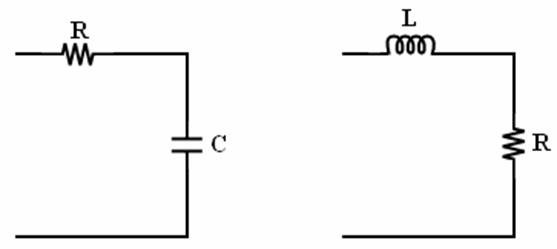# Converting the XBee PWM to an analog voltage for DAC (Digital to Analog Conversion)

The XBee 802.15.4 (Series 1) radio supports Analog Line Passing, this allows you to pass an analog voltage wirelessly without the need for an external microcontroller. The 802.15.4 XBee has an ADC, but does not have a DAC. In order to provide an equivalent analog voltage, the XBee produces a PWM signal.

Below is a simple circuit for converting the XBee/XBee-PRO PWM (Pulse Width Modulation) output to an analog voltage. If the PWM signal is ran through a low-pass filter the original analog voltage can be recovered. The easiest method for this is to make either a RL (Resistor Inductor) or RC (Resistor Capacitor) low-pass filter.

### RL Filter?c = 1/RC fc = 1/(2?RC) ?c = R/L fc= R/(2?L)

The values that are selected for the LPF can be calculated from the cutoff frequency for the filters with the above equations. Since the output of the LPF is going to be DC a relatively low cutoff frequency can be used.

A cutoff frequency of 187Hz leaves a good amount of room for intentional AC signals to make it through the LPF while significantly attenuating the PWM.

Example:

RC low-pass filter:

fc = 187Hz

C = 1?F

R = 850?

Note: Because the analog voltage is converted to a digital value via ADC and then recovered through a filter, the output voltage will not exactly match the input voltage. Please allow for some level of inaccuracy.

Last updated: Aug 08, 2017

### Recently Viewed

No recently viewed articles